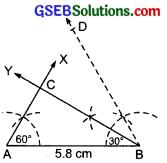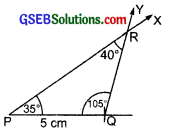# GSEB Solutions Class 7 Maths Chapter 10 Practical Geometry Ex 10.4

Gujarat Board GSEB Textbook Solutions Class 7 Maths Chapter 10 Practical Geometry Ex 10.4 Textbook Questions and Answers.

## Gujarat Board Textbook Solutions Class 7 Maths Chapter 10 Practical Geometry Ex 10.4Question 1.
Construct ∆ABC, given m∠A = 60°, m∠B = 30° and AB = 5.8 cm.
Solution:
Steps of construction:
I. Draw a line segment AB = 5.8 cm.
II. Construct ∠BAX = 60° at A.
III. At B, construct ∠ABY = 30°.
IV. Let the rays AX and BY intersect at C.Thus, ∆ABC is the required triangle.Question 2.
Construct ∆PQR if PQ = 5 cm, m∠PQR = 105° and m∠QRP = 40°.
(Hint: Recall angle-sum property of a triangle).
Solution:
Here the line segment PQ is given. We can construct the triangle, if the measure of ∠QPR is known.
Here, ∠QPR = 180° – [∠PQR + ∠QRP]
= 180° – [105° + 40°]
= 180° – [145°] = 35°
Steps of construction:
I. Draw a line segment PQ = 5 cm.
II. At P, construct ∠QPX = 35°.
III. At Q, construct ∠PQY = 105°.
IV. Let the rays $$\overrightarrow{\mathrm{PX}}$$ and $$\overrightarrow{\mathrm{QY}}$$ intersect at R.Thus, ∆PQR is the required triangle.Question 3.
Examine whether you can construct ∆DEF such that EF = 7.2 cm, m∠E =110° and m∠F = 80°. Justify your answer.
Solution:
Here, m∠E = 110° and m∠F = 80°.
i.e., m∠E + m∠F = 110° + 80° = 190° > 180°. Since, sum of the three angles of a triangle is 180°.
∴ The ADEF is not possible, having sum of two angles > 180°.
Thus, ∆DEF cannot be constructed.